# 1D Property Section TypesYou will find here information about the 1D property section type (also available with the local 1D properties).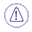• Make sure a material was applied to the geometry and a linear 1D mesh part was assigned to the beam (it is already done in this particular case).
To know more about linear 1D mesh part, refer to Creating 1D Mesh Parts in this guide.

• You cannot apply 1D properties and 1D mesh parts on geometry included in a sketch.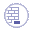Open the sample52.CATAnalysis document from the sample directory.You can select different section types.
For each Type option, you have to click the Component Edition icon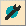in the 1D Property dialog box to define the parameters of the section.

Type: lets you choose the type of section (and symbol) and define the parameters.

 Cylindrical beam: Radius: R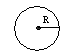Tubular beam: Outside Radius: Ro Inside Radius: Ri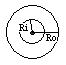Rectangular beam: Length (Y): L Height (Z): H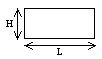Thin Box beam: Exterior Length (Y): Le Exterior Height (Z): He Interior Length (Y): Li Interior Height (Z): Hi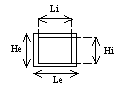Thin U-beam: Global Length (Y): L Global Height (Z): H Global Thickness: T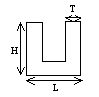Thin I-beam: Global Length (Y): L Associated Thickness: Tl  Global Height (Z): H Associated Thickness: Th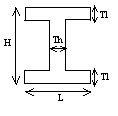Thin T-beam: Global Length (Y): L Associated Thickness: Tl  Global Height (Z): H Associated Thickness: Th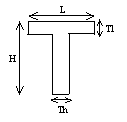Thin X-beam: Global Length (Y): L Associated Thickness: Tl  Global Height (Z): H Associated Thickness: Th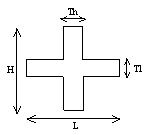User-defined beam: Cross-sectional Area (A) Ixx Iyy Izz Shear center (Y) Shear center (Z) Shear Factor (XY) Shear Factor (XZ) Any section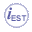Beam from surface: Arbitrary section Compute and display Cross-sectional Area (A) Ixx Iyy Izz Shear center (Y) Shear center (Z) Shear Factor (XY) Shear Factor (XZ) Any surface section*Bar: Cross-sectional Area (A) Any section which works in traction/strain*Variable beam: Start: Cross-sectional Area (A) Ixx Iyy Izz Shear Factor (XY) Shear Factor (XZ) End: Cross-sectional Area (A) Ixx Iyy Izz Shear Factor (XY) Shear Factor (XZ) Any section at each extremities (at each extremities, the center of gravity and the shear center must be coincident)

Where :

• Ixx, Iyy and Izz are the three components of the inertia matrix of the beam, expressed in the principal axis of the beam (axis in which the matrix is diagonal):
• Ixx is the torsion coefficient.
• Iyy is the area moment of inertia in the XZ-plane.
• Izz is the area moment of inertia in the XY-plane.
• Shear center (Y): is the y-coordinate of the shear center in the section axis, centered at the center of gravity of the section.
• Shear center (Z): is the z-coordinate of the shear center in the section axis, centered at the center of gravity of the section.
• Shear Factor (XY): is the ratio between the cross-sectional area (A) and the shear area (Ay), area section which works in shearing in the XY-plane (case of a short beam). As defined, this ratio should be superior to one.
Shear Factor (XY) = A / Ay
As defined, this ratio should be superior to one.
• Shear Factor (XZ): is the ratio between the cross-sectional area (A) and the shear area (Az), area section which works in shearing in the XZ-plane (case of a short beam).
Shear Factor (XZ) = A / Az
As defined, this ratio should be superior to one.*: Beam from surface option: The surface must be a 2D feature (as Fill, Join, ...). You cannot select a sketch. The surface must be plane and continue. Bar option: Before launching a Mesh only or All computation with this option, make sure that the mesh element type is bar. For more details, refer to Changing Element Type.